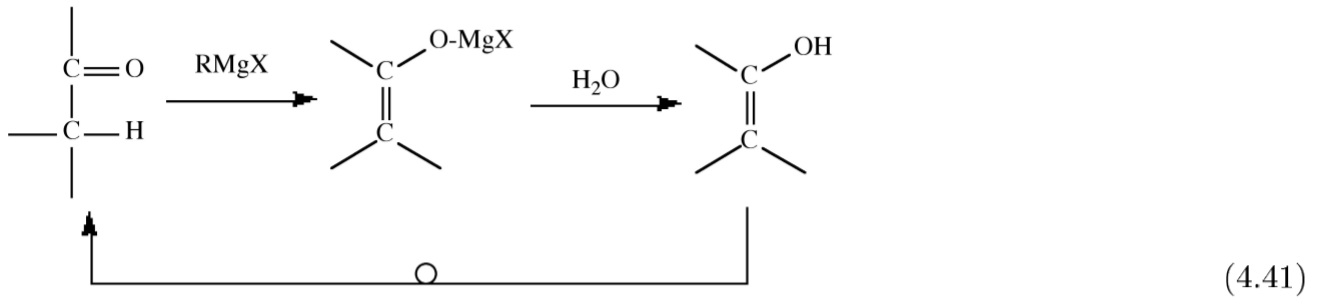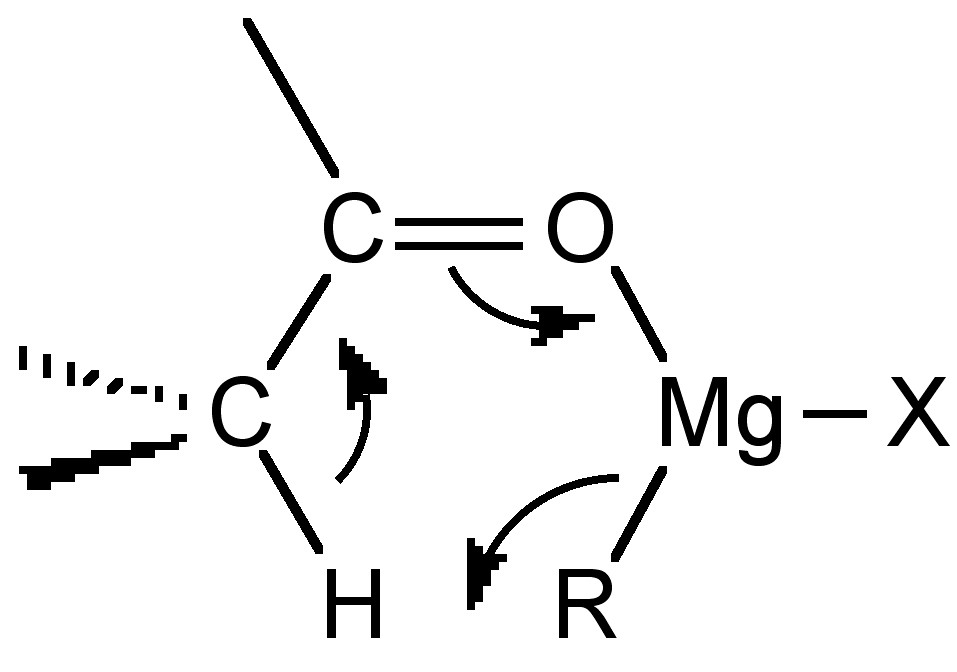# 4.4: Organometallic Compounds of Magnesium

$$\newcommand{\vecs}{\overset { \rightharpoonup} {\mathbf{#1}} }$$ $$\newcommand{\vecd}{\overset{-\!-\!\rightharpoonup}{\vphantom{a}\smash {#1}}}$$$$\newcommand{\id}{\mathrm{id}}$$ $$\newcommand{\Span}{\mathrm{span}}$$ $$\newcommand{\kernel}{\mathrm{null}\,}$$ $$\newcommand{\range}{\mathrm{range}\,}$$ $$\newcommand{\RealPart}{\mathrm{Re}}$$ $$\newcommand{\ImaginaryPart}{\mathrm{Im}}$$ $$\newcommand{\Argument}{\mathrm{Arg}}$$ $$\newcommand{\norm}{\| #1 \|}$$ $$\newcommand{\inner}{\langle #1, #2 \rangle}$$ $$\newcommand{\Span}{\mathrm{span}}$$ $$\newcommand{\id}{\mathrm{id}}$$ $$\newcommand{\Span}{\mathrm{span}}$$ $$\newcommand{\kernel}{\mathrm{null}\,}$$ $$\newcommand{\range}{\mathrm{range}\,}$$ $$\newcommand{\RealPart}{\mathrm{Re}}$$ $$\newcommand{\ImaginaryPart}{\mathrm{Im}}$$ $$\newcommand{\Argument}{\mathrm{Arg}}$$ $$\newcommand{\norm}{\| #1 \|}$$ $$\newcommand{\inner}{\langle #1, #2 \rangle}$$ $$\newcommand{\Span}{\mathrm{span}}$$$$\newcommand{\AA}{\unicode[.8,0]{x212B}}$$

While beryllium makes a range of organometallic compounds, their hazardous nature has limited their study. In contrast, the ionic nature of calcium, strontium, and barium compounds limits the number of stable organometallic derivatives. However, the organometallic chemistry of magnesium is rich and extensive. The importance of Grignards (RMgX, where X = halide) and dialkyl magnesium compounds (R2Mg) is due to their use in organic synthesis and as synthons for a range of organometallic compounds.

## Grignard reagents

Grignard reagents (and the Grignard reaction using these compounds) are named after Victor Grignard (Figure $$\PageIndex{4}$$.45). After studying mathematics at Lyon he transferred to chemistry, becoming a professor at the University of Nancy in 1910. During World War I, he was involved in the eld of chemical warfare; however, it is for his major contribution to organic chemistry he is remembered.Figure $$\PageIndex{4}$$.45: French chemist and Nobel Prize winner François Auguste Victor Grignard (1871 1935).

### Preparation

The general the synthesis of a Grignard reagent involves the reaction of an alkyl halide (RX, where X = Cl, Br, I) with magnesium metal in a suitable ether solvent, (4.4.1).

$RX + Mg \rightarrow RMgX$

While diethyl ether (Et2O) and tetrahydrofuran (THF) are commonly used as solvents, other polar nonprotic solvents are suitable, including: triethylamine (NEt3), dimethylsulphide (Me2S), dimethylselenide (Me2Se), and dimethyltelluride (Me2Te).

In general the alkyl halide is added to an excess of magnesium suspended in the solvent. In most cases it is necessary to activate the magnesium, by the addition of iodine (I2), 1,2-dibromoethane, or sonication. If the halide is very inert reaction can be promoted by the co-condensation of magnesium and THF under vacuum.

There is often an induction period after the initial addition of alkyl halide. However, since the reaction, (4.4.1), is highly exothermic care should be taken to ensure that the reaction does not run-away. For this reason it is normal to initially add a small quantity of the alkyl halide to ensure the reaction initiates. Once reaction is initiated, the addition of alkyl halide is maintained at a suitable rate to ensure the reaction is maintained until all the alkyl halide is consumed. The excess reaction magnesium is removed from the reaction mixture by filtration.

It is not always necessary to use a liquid or solid halide dissolved in the solvent. Bubbling methyl chloride (MeCl) through an Et2O suspension of magnesium yields MeMgCl. The advantage of a gaseous alkyl halide is that the reaction is very clean as all the magnesium is consumed and the excess alkyl halide is bubbled away.

The purity of the magnesium is very important. For his original experiments Grignard used magnesium of a purity of 99.2%. However, it is now more typical to use 99.8% pure magnesium. It is important that the magnesium not be too pure since it is thought that the transition metal impurities catalyze the reaction.

The relative order of reactivity of the alkyl halide follows the trend:

$I > Br > Cl > F$

In fact alkyl uorides are suciently inert that highly coordinating polar solvents such as THF or dimethylformamide (DMF) must be used.

If the reaction is allowed to get too hot then several possible side reactions can occur. In THF reaction with the solvent occurs:

$RMgX + THF \rightarrow RH + H_2C=CH_2 + H_2C=C(H)MgX$

Alternatively, a transition metal catalyzed radical coupling between the Grignard and unreacted alkyl halide is observed irrespective of the identity of the solvent, (4.4.4).

$RMgX + RX \rightarrow R-R + MgX_2$

The mechanism for Grignard formation is thought to be radical in nature; however, a study of the surface of the magnesium during the reaction has shown the presence of corrosion pits. It is generally agreed that initiation occurs at surface dislocations, but the major reaction occurs at a polished surface.

The kinetics of the reaction is 1st order with respect to the alkyl halide concentration, but it has also been claimed to be 1st order with respect to the solvent concentration. It has therefore been concluded that the rate-determining step involves the metal solvent interface.

The reaction of magnesium with aryl bromides has been studied and is proposed to occur by two reactions. The first involves electron transfer between the aryl halide and the metal, while the second involves aryl radical formation.A number of alternative synthetic routes are used with polyhalogenated hydrocarbons, (4.4.5) and (4.4.6), and where the alkyl radical is unstable, (4.4.7).

$X_3CH + ^iPrMgX \rightarrow (X_3C)MgX + ^iPrH$

$C_6Br_6 + EtMgX \rightarrow (C_6Br_5)MgX + EtBr$

$RX + R'MgX' \rightarrow RMgX' + R'X$

### Structure

The solid state structure of Grignard reagents is controlled by the presence and identity of the solvent used in the synthesis. In this regard the size and the basicity of the solvent is important. For example, the structure of EtMgBr crystallized from diethyl ether exists as a 4-ccordinate monomer (Figure $$\PageIndex{4}$$.46a), while the use of the sterically less demanding THF results in a 5-coordinate monomeric structure (Figure $$\PageIndex{4}$$.46b). In contrast, the use of triethylamine yields a dimeric bromide bridged structure (Figure $$\PageIndex{4}$$.46c), and the use of a chelate bidentate amine gives a structure (Figure $$\PageIndex{4}$$.46d) similar to that observed with diethyl ether (Figure $$\PageIndex{4}$$.46a).Figure $$\PageIndex{4}$$.46: Molecular structure of EtMgBr in (a) diethyl ether, (b) THF, (c) triethyl amine, and (d) tetramethyletheylenediamine (TMED).

In solution, Grignards are fluxional such that no single defined structure is present. The series of exchange reactions are known as an extended Schlenk equilibrium (Figure $$\PageIndex{4}$$.47).Figure $$\PageIndex{4}$$.47: Schematic representation of the extended Schlenk equilibrium observed for Grignard compounds in solution.

It is observed that Grignard solutions are also slightly conducting, and magnesium is deposited at both the anode and cathode suggesting the formation of RMg+ and [RMgX2]-. The alkyl/halide exchange is thought to occur through a bridging intermediate (Figure $$\PageIndex{4}$$.48).Figure $$\PageIndex{4}$$.48: Proposed structure for the alkyl/halide exchange bridging intermediate.

## Dialkyl magnesium (R2Mg)

Dialkyl magnesium compounds are involatile white solids. They generally have similar reactivity to their Grignard analogs.

### Synthesis

The most common synthesis of R2Mg is by the reaction of a Grignard with dioxane (C4H8O2), (4.32), where the precipitation of the dihalide is the reaction driving force.This method is useful for the synthesis of cyclic compounds, (4.33).An alternative synthesis that does not require dioxane involves the metal exchange reaction between magnesium metal and a dialkyl mercury compound.

$R_2Hg + Mg \rightarrow R_2Mg + Hg$

Finally, in selected cases, magnesium will react with acidic hydrocarbons such as cyclopentadienyl at high temperatures (600C).

### Structure

In the vapor phase dialkyl magnesium compounds are generally monomeric linear compounds. In solution, in the absence of coordinating solvents R2Mg form a variety of oligomers (Figure $$\PageIndex{4}$$.49a-c) in solution as determined by molecular weight measurements. In the presence of coordinating solvents 4-coordinate monomers predominate (Figure $$\PageIndex{4}$$.49d).Figure $$\PageIndex{4}$$.49: Solution structure of R2Mg (R = Me, Et) in (a c) non-coordinating solvents, and (d) diethyl ether.

As similar trend is observed in the solid state, where polymers have been characterized in the absence of coordinating solvents (Figure $$\PageIndex{4}$$.50a), while monomers or dimmers are generally observed when crystallized from a coordinating solvent (Figure $$\PageIndex{4}$$.50b and c).Figure $$\PageIndex{4}$$.50: Solid state structure of R2Mg (R = Me, Et) crystallized in (a) the absence and (b and c) the presence of a coordinating solvents.

## The use of organomagnesium compounds in organic synthesis

### Hydrolysis and related reactions

Grignard compounds react with water to give the hydrocarbon, (4.4.9), they also react with other hydroxylic compounds such as alcohols and carboxylic acids. One important use of the hydrolysis reaction is specifically deuteration, (4.4.10).

$CH_3MgBr + H_2O \rightarrow CH_4 + BrMgOH$

$CH_3CH_2(CH_3)_2CMgBr + D_2O \rightarrow CH_3CH_2(CH_3)_2CD + BrMgOD$

The hydrogen atom on a terminal alkyne is suciently acidic that the reaction with Grignards occurs in an analogous manner to that of hydrolysis.

$C_6H_5C=CH + C_2H_5MgBr \rightarrow C_6H_5C=CMgBr + C_2H_6$

Once formed the alkynyl Grignard undergoes the same hydrolysis reaction.

$C_6H_5C=CMgBr + D_2O \rightarrow C_6H_5C=CD + BrMgOD$

### Reaction with CO2

Grignards react readily with carbon dioxide to form the carboxylate, which yields the associated carboxylic acid upon hydrolysis, (4.4.13).

$RMgX + CO_2 \rightarrow RCO_2MgX \xrightarrow{H_2O} RCO_2H + HOMgX$

### Reaction with carbonyls

Organomagnesium compounds react with organic carbonyls (aldehydes, ketones, and esters) to yield the alcohol on hydrolysis, (4.4.14). This synthetic route is useful for the formation of primary, secondary and terminal alcohols.

$RMgX + R'_2C=O \rightarrow R'_2(R)COMgX \xrightarrow{H_2O} R'_2(R)COH + HOMgX$

Unfortunately, for some carbonyls there is a competing side reaction of enolization, where the starting ketone is reformed upon hydrolysis.When the Grignard reagent has a β-hydrogen another side reaction occurs in which the carbonyl group is reduced and an alkene is formed.

$R_2C=O + (CH_3CH_2)MgX \rightarrow R_2(H)COH + H_2C=CH_2$

Both the enolization and reduction occur via similar 6-membered cyclic transition states (Figure $$\PageIndex{4}$$.51).Figure $$\PageIndex{4}$$.51: Representation of the 6-membered transition state reaction for enolization of a ketone.

Grignards react with α,β-unsaturated ketones to give either the 1,2-addition product or the 1,4-addition product, or both.

$\text{Ph(H)C=C(H)-C(O)Me + EtMgBr} \xrightarrow{H_2O} \text{Ph(H)C=C(H)-C(OH)EtMe} + \text{Ph(H)EtC-CH}_2\text{-C(O)Me}$

#### Reaction with acyl halides

Acyl halides react with Grignards to give ketones (4.4.16). Best results are obtained if the reaction is carried out at low temperature and in the presence of a Lewis acid catalysts (e.g., (FeCl3).

$\text{CH}_3\text{C(O)Cl + RMgX} \rightarrow \text{CH}_3\text{C(O)R + XMgCl}$

#### Reaction with epoxides

Oxirane (epoxide) rings are opened by Grignards in a useful reaction that extends the carbon chain of the Grignard by two carbon atoms. This reaction is best performed with ethylene oxide since the magnesium halide formed is a Lewis acid catalyst for further reactions in the case of substituted oxiranes.#### Reaction with salts

One of the most useful methods of preparing organometallic compounds is the exchange reaction of one organometallic compound with a salt of a different metal, Equation. This is an equilibrium process, whose equilibrium constant is defined by the reduction potential of both metals. In general the reaction will proceed so that the more electropositive metal will form the more ionic salt (usually chloride).

$\text{RM + M'X} \leftrightharpoons \text{RM' + MX}$

Grignard reagents are particularly useful in this regard, and may be used to prepare a wide range of organometallic compounds. For example:

$\text{2 CH}_3\text{CH}_2\text{MgCl + CdCl}_2 \rightarrow \text{Cd(CH}_2\text{CH}_3\text{)}_2 \text{ + 2 MgCl}_2$

$\text{4 CH}_3 \text{MgCl + SiCl}_4 \rightarrow \text{Si(CH}_3\text{)}_4 \text{ + 4 MgCl}_2$

The reaction with a Grignard is milder than the analogous reaction with lithium reagents, and leads to a lower incident of side-products.

### Bibliography

• H. Bader and N. M. Smyth, J. Org. Chem., 1964, 29, 953.
• C. L. Hill, J. B. Vander Sande, G. M. Whitesides, J. Org. Chem., 1980, 45, 1020.
• E. Weiss, J. Organomet. Chem., 1964, 2, 314.
• A. R. Barron, J. Chem. Soc., Dalton Trans., 1989, 1625.

This page titled 4.4: Organometallic Compounds of Magnesium is shared under a CC BY 3.0 license and was authored, remixed, and/or curated by Andrew R. Barron (CNX) via source content that was edited to the style and standards of the LibreTexts platform; a detailed edit history is available upon request.